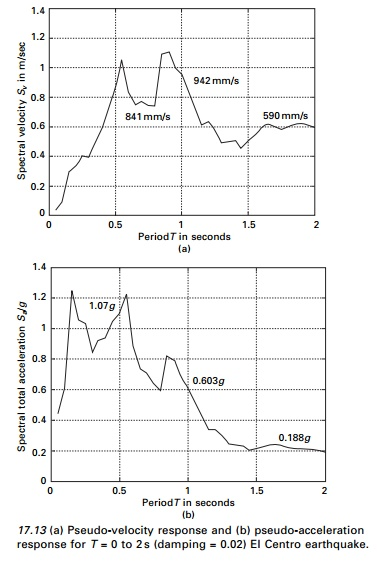Home | | Structural Dynamics and Earthquake Engineering | Response spectrum concept, Pseudo-velocity spectrum

# Response spectrum concept, Pseudo-velocity spectrum

G W Housner was instrumental in the widespread acceptance of the concept of earthquake response spectrum introduced by M A Biot in 1932.

Response spectrum concept

G W Housner was instrumental in the widespread acceptance of the concept of earthquake response spectrum introduced by M A Biot in 1932. This is apractical way of characterizing the ground motions and their effects with structure. It provides a convenient means of summarizing peak response of all possible SDOF systems to a particular component of ground motion. It also provides a practical approach to apply knowledge of dynamics to design of structure and development of lateral force requirements in loads. We have already seen that

In typical engineering structure the percentage of critical damping is relatively small. It is approximately 2â€“8% for steel buildings and 5â€“10% for concrete structures.One can plot deformation with respect to natural period for the El Centro earthquake as shown in Fig. 17.12. The pseudo-velocity and pseudo-acceleration are also plotted in Fig. 17.13a.

Pseudo-velocity spectrum

Consider spectral velocity related to deformation asV = velocity = Ď‰nD Let peak deformation

D â‰ˇ u0 17.19b Then elastic force is obtained from the strain energy or kinetic energy of the mass.The right-hand side is the kinetic energy of mass with velocity V and is called peak pseudo-velocity.

A pseudo-spectral velocity response spectrum is a plot of V in terms of natural period Tn.

Spv = Ď‰Sd           --- --- 17.20

Study Material, Lecturing Notes, Assignment, Reference, Wiki description explanation, brief detail
Civil : Structural dynamics of earthquake engineering : Response spectrum concept, Pseudo-velocity spectrum |